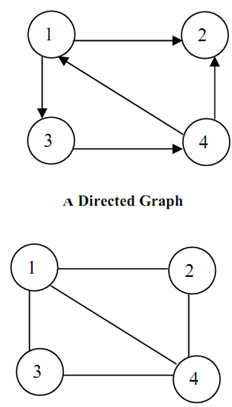## Adjacency matrix of an undirected graph, Data Structure & Algorithms

Assignment Help:

1) What will call a graph that have no cycle?

2) Adjacency matrix of an undirected graph is------------- on main diagonal.

3) Represent the following graphs by adjacency matrix:A  Graph

#### Write an algorithm inputs speed of cars using pseudocode, Write an algorith...

Write an algorithm by using pseudocode which: Inputs top speeds of 5000 cars Outputs fastest speed and the slowest speed Outputs average speed of all the 5000 cars

#### Amortized algorithm analysis, In the amortized analysis, the time needed to...

In the amortized analysis, the time needed to perform a set of operations is the average of all operations performed. Amortized analysis considers as a long sequence of operations

#### Comp. sci algorithms, 1. develop an algorithm which reads two decimal numbe...

1. develop an algorithm which reads two decimal numbers x and y and determines and prints out wether x>y or y>x. the input values, x and y, are whole number > or equal to 0, which

#### Procedure to delete all terminal nodes of the tree, Q. Let a binary tree 'T...

Q. Let a binary tree 'T' be in memory. Write a procedure to delete all terminal nodes of the tree.       A n s . fun ction to Delete Terminal Nodes from Binary Tree

#### Write the algorithm of the quick sort, Ans. An algorithm for the quick...

Ans. An algorithm for the quick sort is as follows: void quicksort ( int a[ ], int lower, int upper ) { int i ; if ( upper > lower ) { i = split ( a, lower, up

#### Algorithm for similar binary tree, Q. The two Binary Trees are said to be s...

Q. The two Binary Trees are said to be similar if they are both empty or if they are both non- empty and left and right sub trees are similar. Write down an algorithm to determine

#### Construction of a binary tree , Q. Construct a binary tree whose nodes in i...

Q. Construct a binary tree whose nodes in inorder and preorder are written as follows: Inorder : 10, 15, 17, 18, 20, 25, 30, 35, 38, 40, 50 Preorder: 20, 15, 10

#### The space - time trade off, The best algorithm to solve a given problem is ...

The best algorithm to solve a given problem is one that requires less space in memory and takes less time to complete its execution. But in practice it is not always possible to

#### COBOL, write a COBOL program to find the biggest of two numbers

write a COBOL program to find the biggest of two numbers

#### Graph, Multilist Representation of graph

Multilist Representation of graph